# Calculate the frequency counts of each unique value of a Pandas series

• Last Updated : 17 Aug, 2020

Let us see how to find the frequency counts of each unique value of a Pandas series. We will use the value_counts() function to perform this task.

Example 1 :

Attention geek! Strengthen your foundations with the Python Programming Foundation Course and learn the basics.

To begin with, your interview preparations Enhance your Data Structures concepts with the Python DS Course. And to begin with your Machine Learning Journey, join the Machine Learning - Basic Level Course

 `# importing the module``import` `pandas as pd`` ` `# creating the series``s ``=` `pd.Series(data ``=` `[``2``, ``3``, ``4``, ``5``, ``5``, ``6``, ``                      ``7``, ``8``, ``9``, ``5``, ``3``])`` ` `# displaying the series``print``(s)`` ` `# finding the unique count``print``(s.value_counts())`

Output :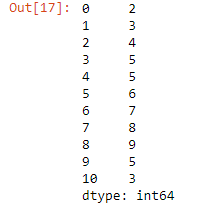Output: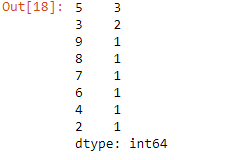Example 2 :

 `# importing the module``import` `pandas as pd`` ` `# creating the series``s ``=` `pd.Series(np.take(``list``(``'0123456789'``), ``              ``np.random.randint(``10``, size ``=` `40``)))`` ` `# displaying the series``print``(s)`` ` `# finding the unique count``s.value_counts()`

Output :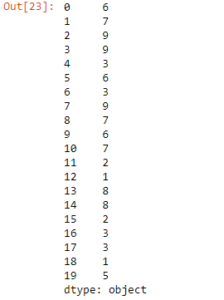Output: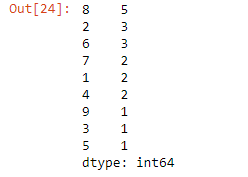Example 3 :

 `# importing pandas as pd ``import` `pandas as pd ``   ` `# creating the Series ``sr ``=` `pd.Series([``'Mumbai'``, ``'Pune'``, ``'Agra'``, ``'Pune'``, ``                ``'Goa'``, ``'Shimla'``, ``'Goa'``, ``'Pune'``]) `` ` `# displaying the series ``print``(sr) `` ` `# finding the unique count``sr.value_counts()`

Output :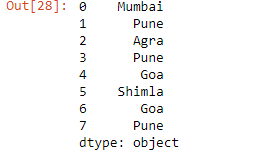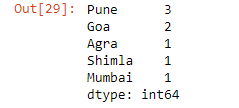My Personal Notes arrow_drop_up